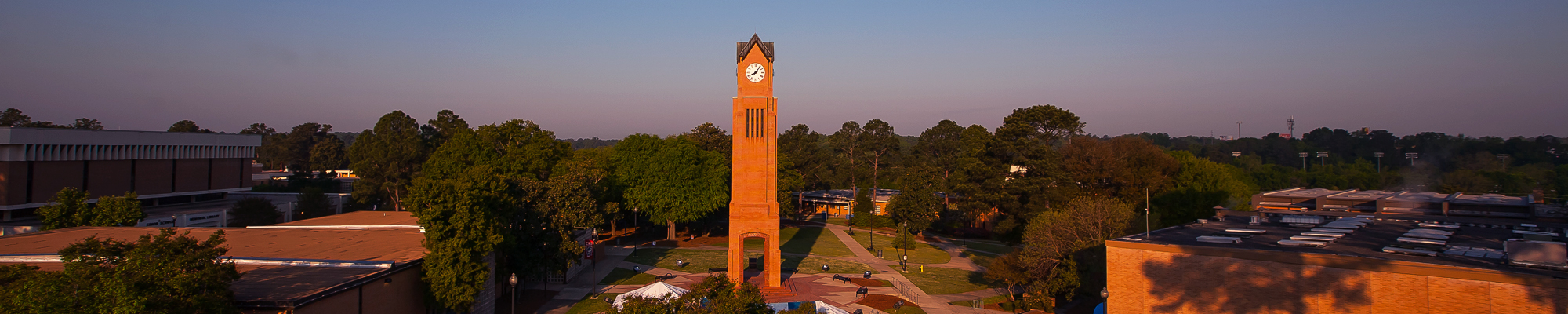## Compare Courses

CPSC 1105. Introduction to Information Technology (3-0-3) This course provides an introduction to computer and information technologies. It discusses the nature of information, computer hardware, software, communications technology, and computer-based information systems. The theory is complemented by practical work aimed at gaining basic proficiency with different types of widely used application software. (Course fee required.)

CPSC 1301. Computer Science 1 (3-0-3) Co-requisite: CPSC 1301L. This course includes an overview of computers and programming; problem solving and algorithm development; simple data types; arithmetic and logic operators; selection structures; repetition structures; text files; arrays (one-and-two-dimensional); procedural abstraction and software design; modular programming (including sub-programs or the equivalent).

CPSC 1301L. Computer Science 1 Lab (0-3-1) Corequisite: CPSC 1301. This course provides a hands-on experience for the students in the area of programming as a realization of the concepts presented in Computer Science 1. The students will complete programming projects using one or more program development environments. The lab projects will apply and reinforce the topics covered in Computer Science 1.

GEOG 2215. Introduction to the Geographic Information Systems (3-2-4) Geography 2215 is the first course in the Geographic Information Systems sequence. This course introduces students to the art and science of Geographic Information Systems (GIS) and related geospatial technologies, geographic analysis, map communication, and geographic inquiry. In the course, students will learn about the major components of Geographic Information Systems, including the hardware, software, people and data needed to make these systems useful.

MATH 1113. Pre-Calculus (4-0-4) Prerequisite: MATH 1111 with a grade of "C" or better or an appropriate math placement score. This course is designed to prepare students for calculus, physics, and related technical subjects. Topics include algebraic and transcendental functions and an intensive study of trigonometric functions.

MATH 1125. Applied Calculus (3-0-3) Prerequisite: MATH 1111 with a grade of "C" or better, MATH 1113 with a grade of "C" or better, or an appropriate math placement score. Introduction to limits and continuity, differential calculus of algebraic, exponential, and logarithmic functions and integration. Applications in the fields of the behavioral, biological, and managerial sciences are included. (Course fee required.)

MATH 1131. Calculus with Analytic Geometry 1 (4-0-4) Prerequisite: MATH 1113 with a grade of "C" or better or an appropriate math placement score. Topics include exponential and logarithmic functions, introduction to limits and derivatives, computation and application of derivatives, and the definite integral. (Course fee required.)

MATH 1132. Calculus with Analytic Geometry 2 (4-0-4) Prerequisite: MATH 1131 with a grade of "C" or better. Topics include the definite and indefinite integrals, improper integrals, techniques of integration, applications of integration, and infinite sequences and series. (Course fee required.)

MATH 1165. Computer-Assisted Problem Solving (3-0-3) Prerequisites: MATH 1125 or MATH 1131 with a grade of "C" or better. Classes may be taken concurrently. Problem solving using contemporary technology such as graphing calculators, spread sheets, and computer algebra systems. Topics may include interpolation; linear regression; elementary differential models; and an introduction to the fundamentals of computer algebra systems, including manipulation of lists, sets, data structures, functions, plots, and program control structures. (Course fee required.)

MATH 2125. Introduction to Discrete Mathematics (3-0-3) Prerequisite: MATH 1113 or MATH 1131 with a grade of "C" or better or a satisfactory math placement score. Topics include logic and proof, sets, functions, algorithms, the integers, matrices, mathematical reasoning, induction, recursion, counting, discrete probability, relations, graphs, trees, and Boolean algebra. (Course fee required.)

PHIL 2500. Formal Logic (3-0-3) An introduction to contemporary techniques in logic with special attention given to deductive models and decision methods. Emphasis is placed on the application of logic to argument analysis, problem solving, foundations of mathematics, science, and computer science.

STAT 1127. Introductory Statistics (3-0-3) Prerequisite: Satisfactory score on math placement exam or completion of any of the following courses with a grade of "C" or better: MATH 1001, MATH 1101, MATH 1111, MATH 1113, MATH 1125, or MATH 1131. Survey of modern statistical methods applicable to behavioral, biological, health and managerial sciences, and education. Organization and analysis of data, probability distributions, sampling distributions, point estimation, confidence interval, hypothesis testing, and regression analysis. (Course fee required.)